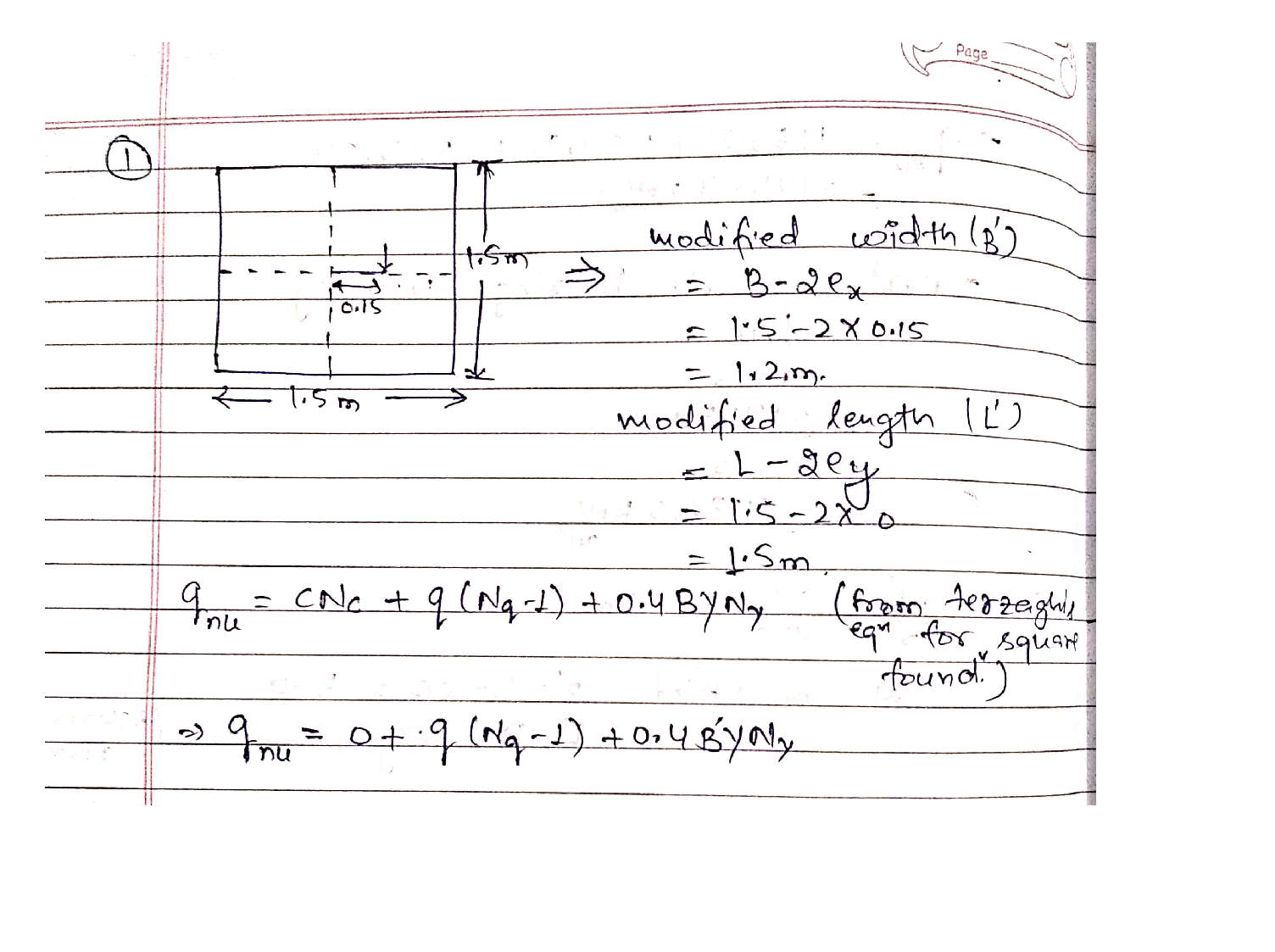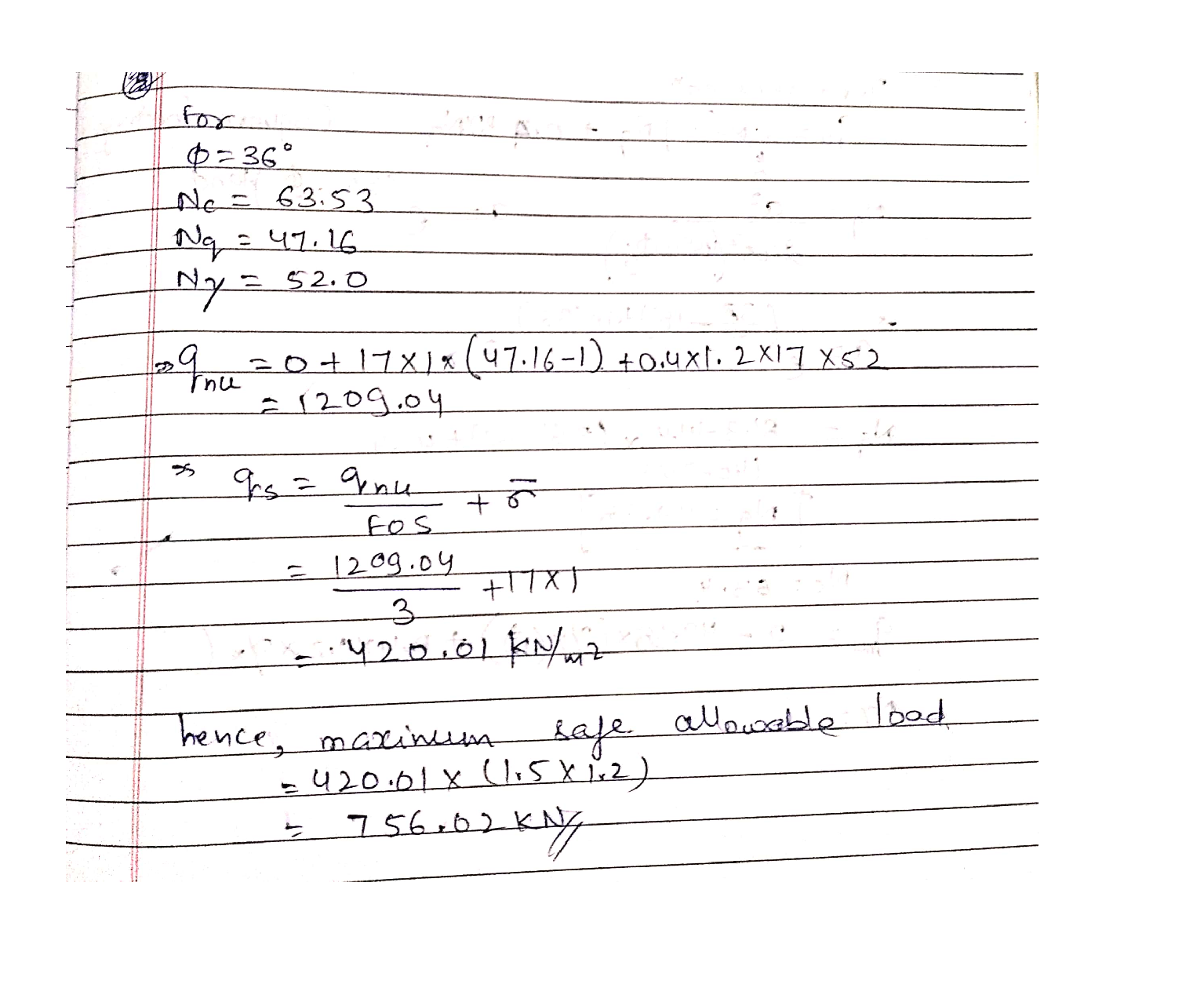Question

Strength of Material

1- An eccentrically loaded foundation is shown in Figure 1. Use FS of 3 and determine the maximum allowable load that the foundation can carry.Verified### Question 44941Strength of Material

For the support of Prob. 1.47, knowing that the diameter of the pins d = 16 mm and that the magnitude of the load is P 20 kN,determine (a) the factor of safety for the pin, (b) the required values of b and e if the factor of safety for the wooden member is the same-as that found in part a for the pin.

### Question 43624Strength of Material

17. Iron has a BCC crystal structure and an atomic radius of 0.1241 nm. Determine the interplanar spacing corresponding to (111) and (211) set of planes. Please look at the previous question to understand what (111) and (211) means.[5 points]

### Question 43623Strength of Material

16. Determine the expected diffraction angle for the first-order reflection (i.e., n=1) from the (310)set of planes for BCC chromium when monochromatic radiation of wavelength 0.0711 nm is-used. (311) means h=3, k=1, and l=1. Please look at the determination of dnki in the slide set corresponding to Lecture 2. Also note that the diffraction angle means 20. You will determine"0" from the equation, and will have to multiply it by 2.[5 points]

### Question 43621Strength of Material

14. Explain the differences between ionic and covalent bonding.

### Question 43620Strength of Material

13. Molybdenum has a BCC crystal structure, an atomic radius of 0.1363 nm, and an atomic weight of 95.94 g/mol. Compute its theoretical density.[3 points]

### Question 43619Strength of Material

12. Calculate the radius of a Palladium atom if it has a FCC crystal structure, a density of 12.0g/cm3 and an atomic weight of 106.4 g/mol.[3 points]

### Question 43617Strength of Material

10. Derive the value for the atomic packing factor of a FCC structure.

### Question 43616Strength of Material

9. In Question 1, it was noted that the net bonding energy En between two isolated positive and negative ions is a function of interionic distance r as follows:
E_{N}=-\frac{A}{r}+\frac{B}{r^{n}}
where A, B, and n are constants for the particular ion pair. This equation is also valid for the bonding energy between adjacent ions in solid materials. The modulus of elasticity "E" is proportional to the slope of the interionic force-separation curve at the equilibrium interionic separation that is is E =kx(df/dr)
Derive an expression for the dependence of the modulus of elasticity on these A, B, and n parameters (for the two-ion system) using the following procedure:
\text { a. Establish a relationship for the force } \mathrm{F} \text { as a function of } \mathrm{r} \text {, realizing that } F=\frac{d E_{N}}{d \mathbf{r}}
b. Now take the derivative dF/dr.
c. Substitute the value of ro obtained in Question 1 in the above expression and determine the expression for the modulus of elasticity “E". [Assume k = 2]

### Question 43615Strength of Material

8. The net potential energy between two adjacent ions, En, may be represented as:
E_{N}=-\frac{A}{r}+\frac{B}{r^{n}}
Calculate the bonding energy Eo in terms of the parameters A, B, and n using the following procedure:
a. Differentiate En with respect to r, and then set the resulting expression equal to zero, since the curve of EN versus r is a minimum at Eo.
b. Solve for r in terms of A, B, and n, which yields ro, the equilibrium interionic spacing.
c. Determine the expression for Eo by substitution of ro into the above equation.

### Question 43614Strength of Material

7. Show that volumetric strain is the sum of strains in three orthogonal directions.

### Submit query